Next: Schrödinger Representation Up: Position and Momentum Previous: Poisson Brackets

# Wavefunctions

Consider a simple system with one classical degree of freedom, which corresponds to the Cartesian coordinate. Suppose thatis free to take any value (e.g.,could be the position of a free particle). The classical dynamical variableis represented in quantum mechanics as a linear Hermitian operator which is also called. Moreover, the operatorpossesses eigenvalues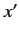lying in the continuous range(since the eigenvalues correspond to all the possible results of a measurement of). We can span ket space using the suitably normalized eigenkets of. An eigenket corresponding to the eigenvalueis denoted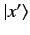. Moreover, [see Equation (85)]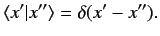(117)

The eigenkets satisfy the extremely useful relation [see Equation (87)]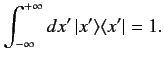(118)

This formula expresses the fact that the eigenkets are complete, mutually orthogonal, and suitably normalized.

A state ket(which represents a general stateof the system) can be expressed as a linear superposition of the eigenkets of the position operator using Equation (118). Thus,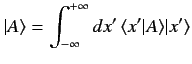(119)

The quantity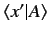is a complex function of the position eigenvalue. We can write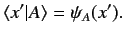(120)

Here,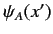is the famous wavefunction of quantum mechanics. Note that stateis completely specified by its wavefunction[because the wavefunction can be used to reconstruct the state ketusing Equation (119)]. It is clear that the wavefunction of stateis simply the collection of the weights of the corresponding state ket, when it is expanded in terms of the eigenkets of the position operator. Recall, from Section 1.10, that the probability of a measurement of a dynamical variable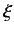yielding the result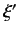when the system is in stateis given by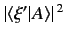, assuming that the eigenvalues ofare discrete. This result is easily generalized to dynamical variables possessing continuous eigenvalues. In fact, the probability of a measurement ofyielding a result lying in the rangeto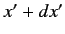when the system is in a stateis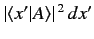. In other words, the probability of a measurement of position yielding a result in the rangetowhen the wavefunction of the system isis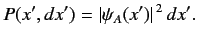(121)

This formula is only valid if the state ketis properly normalized: i.e., if. The corresponding normalization for the wavefunction is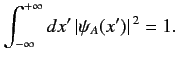(122)

Consider a second staterepresented by a state ket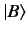and a wavefunction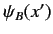. The inner productcan be written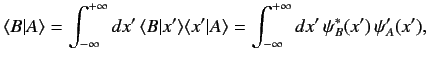(123)

where use has been made of Equations (118) and (120). Thus, the inner product of two states is related to the overlap integral of their wavefunctions.

Consider a general function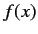of the observable[e.g.,]. If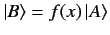then it follows that(124)

giving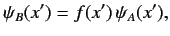(125)

where use has been made of Equation (117). Here,is the same function of the position eigenvaluethatis of the position operator: i.e., ifthen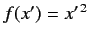. It follows, from the above result, that a general state ketcan be written(126)

where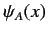is the same function of the operatorthat the wavefunctionis of the position eigenvalue, and the ket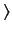has the wavefunction. The ketis termed the standard ket. The dual of the standard ket is termed the standard bra, and is denoted. It is easily seen that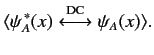(127)

Note, finally, thatis often shortened to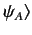, leaving the dependence on the position operatortacitly understood.Next: Schrödinger Representation Up: Position and Momentum Previous: Poisson Brackets
Richard Fitzpatrick 2013-04-08